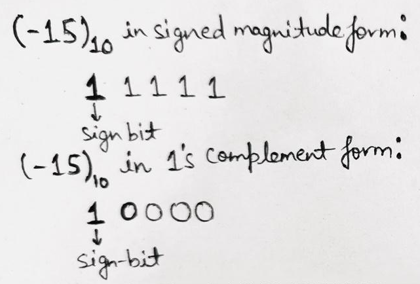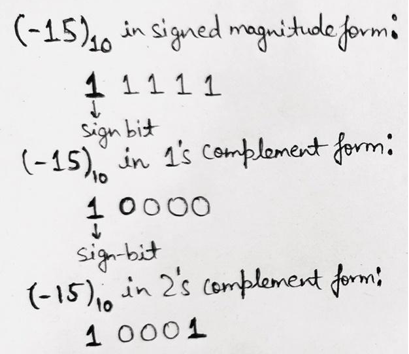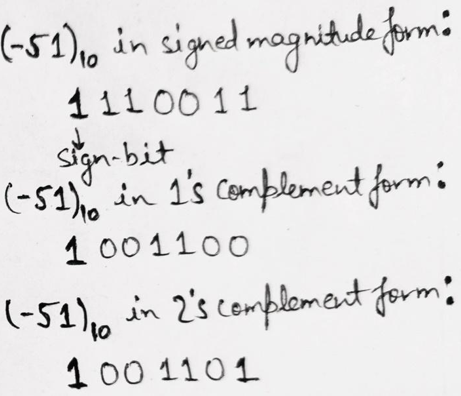# Signed Representation of Binary Numbers

Binary numbers (signed representation): In this tutorial, we will learn about the signed representation of binary numbers with the help of examples. By Saurabh Gupta Last updated : May 10, 2023

Prerequisite: Number systems

Until now, we have only talked about positive numbers and have already discussed their mathematical operations. But there also exists negative numbers in the number system, in this article we are going to learn about how to represent negative numbers in the binary format?

## What are Signed Numbers?

In decimal number system, we have (+) sign to represent the positive number and (-) sign to represent a negative number. But in digital logic, we have two symbols 0 and 1, so we use these symbols to represent the sign of the number.

There are two ways of representing signed numbers,

1. Signed Magnitude Form
2. Complement Form

### 1. Signed Magnitude Form

In signed magnitude form, an additional bit is placed to the extreme left (MSB) of the number to represent the sign; thus, it is known as the sign bit.

We use 0 to represent the positive numbers and 1 to represent the negative numbers. The extra bit is isolated from the magnitude of binary numbers using a comma.

Under the signed-magnitude system, a great amount of manipulation is required to add a positive number to a negative number. Thus, representation is possible but still, it is impractical in nature.

#### Solution

``` (13)10 = (0,1101)2   sign-bit 0 is used to represent the positive number
(-13)10 = (1,1101)2  sign-bit 1 is used to represent the negative number
```

### 2. Complement Form

Since performing arithmetic operations using a signed magnitude form is very complex. Digital computers use the complement form to perform these calculations.

There are two complement forms - 1's complement form and 2's complement form.

If the number is positive then, the magnitude is represented in its true binary form and a sign bit 0 is added to the LHS of the MSB. For a positive number, the representation is the same in signed magnitude, 1's and 2's complement form.

The advantage of using a complement form to perform arithmetic operations is the reduction in the hardware. Instead, of having separate hardware for addition and subtraction only additional adders are needed.

#### 2.1. 1's Complement Representation

The 1's complement of a number can be obtained by replacing each "0 bit with 1 bit" and "1 bit with 0 bit" in the binary number.

##### Solution

(15)10 in binary form can be represented as (1111)2.

Now, to represent its negative sign, we will add a sign bit 1.

Thus, (-15)10 = (1,1111)2. To represent it in 1's complement form, we will replace each 1 with 0 (excluding the sign bit, because if we replace sign bit then its sign will be changed to positive which is not correct). Therefore,#### 2.2. 2's Complement Representation

We can get the 2's complement of a number by finding the 1's complement of number and adding 1 to the LSB of the respective 1's complement.

##### Solution

As we have discussed above, the 1's complement representation of (-15)10 is given as 10000. Now, we have to add a bit 1 to the LSB in the above 1's complement form i.e., we will get,Which is the required 2's complement representation of (-15)10.

##### Solution